# Problems of the Week

Contribute a problem

# 2018-05-28 Intermediate

$A^2 + B^2$, $AB$, and $A + B$ are all integers.

Do both $A$ and $B$ have to be integers?

Six straight, zigzagging lines are drawn inside an $8\times1$ rectangle. The drawing starts at the top left vertex and ends at the top right vertex.

What is the minimum possible sum of the lengths of these 6 segments?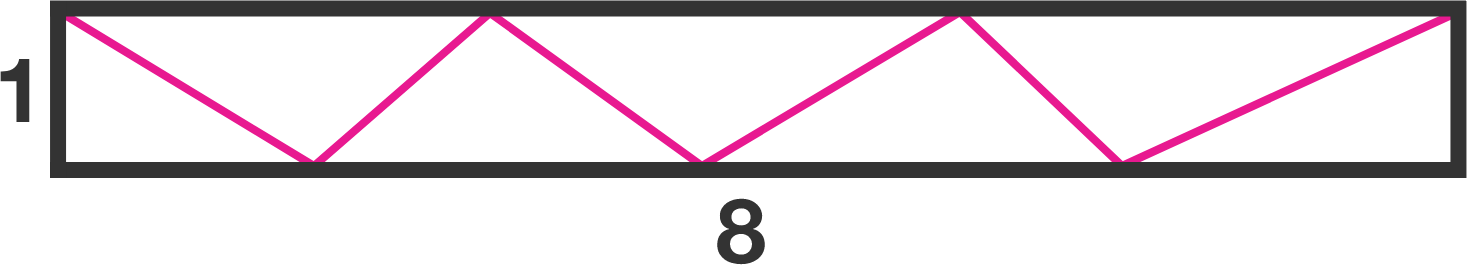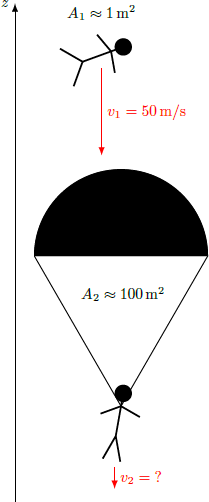Without opening his parachute, a skydiver reaches a fall velocity of $v_1 = 50\text { m/s}.$ When he does open the parachute, he's braked by additional air resistance. After a while, he finally arrives at the ground.

At what fall velocity $v_2$ does he reach the ground?

Details and Assumptions:

• The air frictional force $F_f = F_f (\rho, A, v)$ depends only on the density of the air $\rho ,$ the cross-sectional area of the skydiver $A,$ and the velocity $v.$ Neglect the effect that the shape of the falling object has on the air frictional force.
• The cross-sectional area without the parachute is $A_1 \approx 1\text { m}^2$ and that with the parachute is $A_2 \approx 100\text{ m}^2.$
• The density of the air is constant.

There is a collection of points inside a unit cube that are closer to the center of the cube than to any of the cube’s vertices.

What is the volume of this 3D region?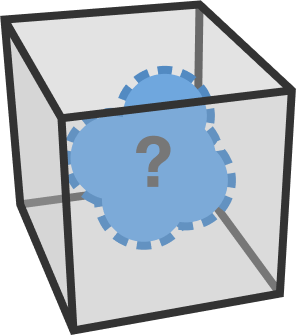The true shape of the 3D region is not shown.

$f(n)$ gives the sum of the cubed digits of some positive integer $n.$ For example, $f(123)=1^3+2^3+3^3=36.$

If we repeatedly apply this process on each previous result, the following two different behaviors may arise:

• Arrive at a fixed point: For example, beginning with 3, we eventually arrive at 153, and $f(153)=153.$ We say that 153 is a fixed point.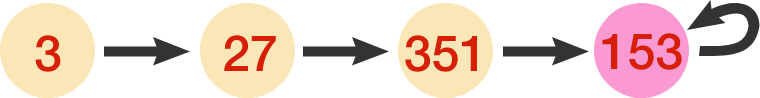• Arrive at a limit cycle: For example, beginning with 4, we eventually arrive at 133, and $f(133) = 55 \implies f(55) = 250 \implies f(250)=133.$ We say that $\{55,133,250\}$ is a limit cycle.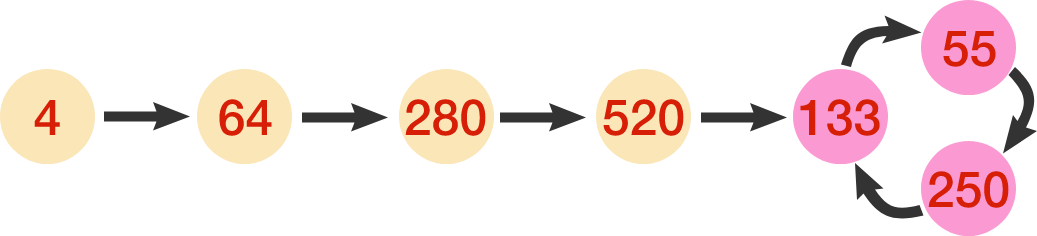Let the limit set be the set of all fixed points and limit cycles in the range of $f(n).$

Find the sum of all the numbers in the limit set (including the four in pink found above). Note: A coding environment is provided below:

You need to be connected to run code

Bonus: Prove that the limit set actually contains finitely many numbers.

×